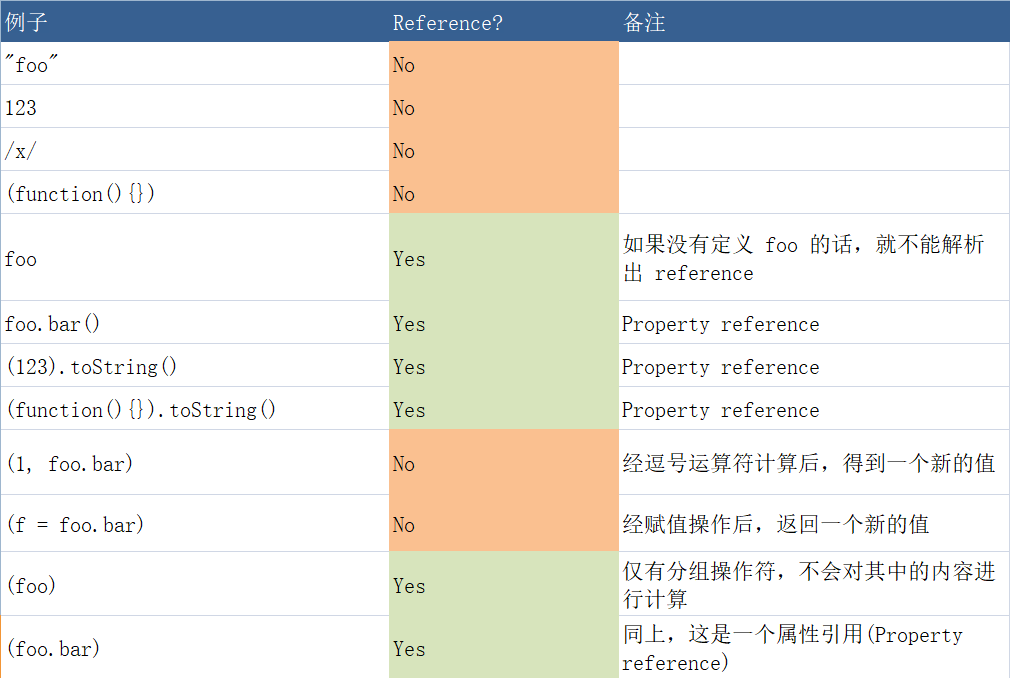# 【JavaScript】从 this 指向到 reference 类型

1）在函数【调用】时，“this”总是指向小数点左侧的那个对象

2）如果没有小数点，那么“this”指向全局作用域（比如 Window，严格模式为 undefined）

3）有几个可以改变“this”指向的函数——bind，call 和 apply

4）关键字 “new” 将 “this” 绑定到那个新创建的对象上

var foo = {bar: function () {return this}}
var b = foo.bar

foo.bar();
b();
var c = new someFunction();


(f = foo.bar)();
(1, foo.bar)();
(foo.bar)();### Reference Specification Type

#### Reference 是什么

ECMAScript 将 Reference 定义为“被解析的命名绑定（resolved name binding）”，它由三部分组成——base,name, and strict flag。

• 标识符解析
• 属性访问'use strict';
var foo;

// 标识符解析会产生 Reference
var Reference = Object.create(null);
Reference.base = EnvironmentRecord;
Reference.name = 'foo';
Reference.strict = true;

// or
foo.bar;

// 属性访问会产生 Reference
var Reference = Object.create(null);
Reference.base = foo;
Reference.name = 'bar';
Reference.strict = true;

// or 使用未声明的变量
a;
var Reference = Object.create(null);
Reference.base = undefined;
Reference.name = 'a';
Reference.strict = true;


### 函数调用

1. Let ref be the result of evaluating MemberExpression.
2. Let func be GetValue(ref).
3. Let argList be the result of evaluating Arguments, producing an internal list of argument values ([see 11.2.4](https://es5.github.io/#x11.2.4)).
4. If Type(func) is not Object, throw a TypeError exception.
5. If IsCallable(func) is false, throw a TypeError exception.
6. If Type(ref) is Reference, then
* If IsPropertyReference(ref) is true, then Let thisValue be GetBase(ref).
* Else, the base of ref is an Environment Record, Let thisValue be the result of calling the ImplicitThisValue concrete method of GetBase(ref).
7. Else, Type(ref) is not Reference.
* Let thisValue be undefined.
8. Return the result of calling the *Call* internal method on func, providing thisValue as the this value and providing the list argList as the argument values.


ES5 标准告诉我们一个事实——只有在函数真正调用的时候，才能判断 this 的值。

### 赋值，逗号和分组操作符

#### 简单赋值(=)操作

1. Let lref be the result of evaluating LeftHandSideExpression.
2. Let rref be the result of evaluating AssignmentExpression.
3. Let rval be GetValue(rref).
4. Throw a SyntaxError exception if the following conditions are all true:
a. Type(lref) is Reference is true
b. IsStrictReference(lref) is true
c. Type(GetBase(lref)) is Environment Record
d. GetReferencedName(lref) is either "eval" or "arguments"
5. Call PutValue(lref, rval).
6. Return rval.

#### 逗号操作符

1. Let lref be the result of evaluating Expression.
2. Call GetValue(lref).
3. Let rref be the result of evaluating AssignmentExpression.
4. Return GetValue(rref).

GetValue 将 foo.bar 的引用转化成那个实际的函数。逗号操作符计算完成后，和调用(function(){})()没有什么分别。

#### 分组操作符

Grouping Operator会使用 GetValue 计算表达式吗？

Return the result of evaluating Expression. This may be of type Reference.
This algorithm does not apply GetValue to the result of evaluating Expression.

（完）

### 参考

know-thy-reference/

posted @ 2019-06-18 11:40  廖飞银  阅读(526)  评论(0编辑  收藏